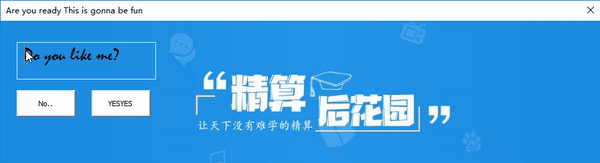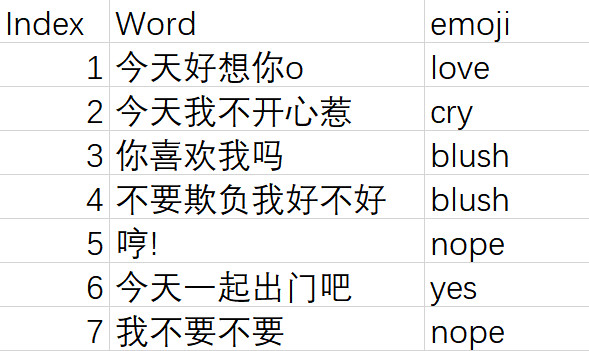# 精算仔秘籍之——如何用 VBA 撩 TA

• 精算仔用 Excel VBA 写的表白利器。只要手里有代码，没有姑娘拿不下！

（文末有彩蛋哦）

珂珂有一个好朋友，小珂仔。

小珂仔是一个可爱聪明善良又胆小的男孩子，他暗恋了一个女生很久，情人节都到了，他还是没有勇气表白，于是向我求助。我指点了他一句话：

只要手里有代码 没有姑娘拿不下

小珂仔恍然大悟，如果能够用 Excel VBA 知识 / 精算知识向喜欢的女孩表白，那该是多么浪漫的事情呀！

于是他写了几个简单的 VBA 程序，以下是演示：第二个图的数据源在这里：但是他突然想到，他最想说的其实是这句话：

你喜欢我吗

如果仅仅用 uniform distribution，无法达到让模拟集中在第三行上的效果。所以，作为一个粗算精算专业的学生，他灵机一动！如果用 Poisson 分布模拟的话，就可以设定模拟均值！

作为小珂仔的好朋友，我决定把写图二VBA 的心路历程记录下来。其它的VBA代码阅读原文可以查看哦。

## 第一步：设置表情包变量

从网上下载emoji 图片，导入excel后把 emoji 图片的名字改为要求的名字。改图片名的操作是，点中图片，看公示栏左边那个地方，默认名字应该是Picture x。在那里你点进去，输入名字，回车。名字已经修改了。

在 VBA 中用如下代码设置变量。（假设 emoji 的名字为 "blush", "yes", "nope", "cry", "love"）

Set blush = Sheet1.Shapes("blush")
Set yes = Sheet1.Shapes("yes")
Set nope = Sheet1.Shapes("nope")
Set cry = Sheet1.Shapes("cry")
Set love = Sheet1.Shapes("love")
Set emoji = Sheet1.Shapes.Range(Array("blush", "yes", "nope", "cry", "love"))


## 第二步：生成Poisson分布随机数

这里介绍三种方法 生成Poisson分布随机数

### 方法 1 Multiplication method

第一种，也是最常见、使用最广泛的一种，叫做 Multiplication method, PM。它最核心的原理是数一个 Poisson process 里面发生事件的数目。作为一个精算专业的学生，小珂仔知道如果 process 发生概率为 1 的话，时间 $t$ 内发生的事件数正好服从均值为 $t$ 的 Poisson 分布。

Public Function RandomPoisson(ByVal lambda As Integer)
r = Exp(-lambda) 'r是e的-t次方
N = 0 'N代表事件个数，也就是我们想要的Poisson分布变量
s = 1  '代表e的 - 经过的时间】次方
Do
N = N + 1
s = s * Rnd() 'U=Rnd()
Loop While s > r '如果经过的时间小于等于t，继续抽样
RandomPoisson = N - 1
End Function


### 方法 2 Sequential search algorithm

第二种，也是精算课本上的方法，叫做 Sequential search algorithm, PS。也就是生成一个 0-1 的随机数，把它对应到 Poisson cumulative distribution 上去，算出其对应的分位数。至此，Poisson 随机数就生成了。核心代码如下：

Public Function RandomPoisson2(lambda As Integer, upperbound As Integer)
Dim DisFreqArray() As Double
Dim CumFreqArray() As Double
ReDim DisFreqArray(upperbound + 1) '设定distribution function
ReDim CumFreqArray(upperbound + 1) '设定cumulative function
'Dim RandNum As Double
DisFreqArray(0) = Exp(-1 * lambda)
CumFreqArray(0) = Exp(-1 * lambda)
For k = 1 To upperbound
DisFreqArray(k) = DisFreqArray(k - 1) * lambda / k
CumFreqArray(k) = CumFreqArray(k - 1) + DisFreqArray(k)
Next k
RandNum = Rnd
k = 1
Do While RandNum > CumFreqArray(k) And k < upperbound + 1
k = k + 1
Loop
RandomPoisson2 = k
End Function


注意，这里我们规定了变量上限以节省空间。
前两种方法最大的缺点是，随着Poisson分布均值的增加，运算速率会变得很慢。

### 方法 3 Data analysis tool

第三种，如果你的excel版本有data analysis tool的话，可以直接用来生成随机数。VBA 里面实现是这样的，其中A, B, C, D, E, F为参数

Application.Run "ATPVBAEN.XLAM!Random", "", A, B, C, D, E, F


参数含义解释如下：

• A = how many variables that are to be randomly generated
• B = number of random numbers generated per variable
• C = number corresponding to a distribution
• 1= Uniform
• 2= Normal
• 3= Bernoulli
• 4= Binomial
• 5= Poisson
• 6= Patterned
• 7= Discrete
• D = random number seed
• E = parameter of distribution (mu, lambda, etc.) depends on choice for C
• (F) = additional parameter of distribution (sigma, etc.) depends on choice for C

但是这种方法的缺点是：

1. 无法直接赋值于 VBA 变量，只能显示在单元格中
2. 不优雅。代码里面带有一个 overwrite alert。如果写入的单元格不是空，那么可能会引发 alert。需要写入代码关掉 alert。

### 第三步：通过随机设定offset的值来实现随机生成对话的效果

Index = RandomPoisson (3) '利用刚刚的函数，生成poisson分布的值
If Index > Up Then Index = Up '设定upper bound
If Index = 0 Then Index = 1 '设定lowerbound
Range("chatbox") = Startchat.Offset(Index, 1) 'Startchat代表数据源里第一个单元格，chatbox代表生成对话的单元格
EmojiIndex = Startchat.Offset(Index, 2)
Select Case EmojiIndex
Case "blush"
EmojiIndex = 1
Case "yes"
EmojiIndex = 2
Case "nope"
EmojiIndex = 3
Case "cry"
EmojiIndex = 4
Case "love"
EmojiIndex = 5
End Select
emoji(EmojiIndex).Visible = True '只让选定的emoji可见


经过努力，小珂仔终于成功向喜欢的女孩表白了！

彩蛋：小珂仔的表白结果